Win up to 100% scholarship on Aakash BYJU'S JEE/NEET courses with ABNAT Win up to 100% scholarship on Aakash BYJU'S JEE/NEET courses with ABNAT

# JEE Main 2022 July 27 – Shift 1 Chemistry Question Paper with Solutions

The JEE Main 2022 July 27 – Shift 1 Chemistry Question Paper with Solutions is given on this page. The JEE Main solutions 2022 can be used by students to check their answers. Those who are planning to appear for the upcoming JEE Main exam can use the JEE Main 2022 question paper with solutions as an ideal study material. Solving the JEE Main Shift 1 July 27 papers will give them an opportunity to find out the topics which they need to work out more. JEE Main 27 July Shift 1 answer key consists of accurate step-by-step solutions. Check out the JEE Main 2022 Chemistry July 27 – Shift 1 Question Paper with Solutions below.

## JEE Main 2022 27th July Shift 1 Chemistry Question Paper and Solutions

SECTION – A

Multiple Choice Questions: This section contains 20 multiple choice questions. Each question has 4 choices (1), (2), (3) and (4), out of which ONLY ONE is correct.

1. 250 g solution of D-glucose in water contains 10.8% of carbon by weight. The molality of the solution is nearest to (Given: Atomic Weights are, H, 1 u; C, 12 u; O, 16 u)

(A) 1.03

(B) 2.06

(C) 3.09

(D) 5.40

Sol. Weight of D-glucose in water = 250 g

∴ Weight of carbon in D-glucose

$$\begin{array}{l}=\frac{250}{180}\times 72 = 100 g \end{array}$$

Percentage of carbon in the aqueous solution of glucose is
= 10.8%

∴ Weight of the solution is = 925.93

∴ Molality of D-glucose is

$$\begin{array}{l}=\frac{\frac{250}{180}}{(925.93-250)}\times 1000\end{array}$$
$$\begin{array}{l}=\frac{250}{180\times 675.93}\times 1000 = 2.06 \end{array}$$

2. Given below are two statements.

Statement I: O2, Cu2+, and Fe3+ are weakly attracted by magnetic field and are magnetized in the same direction as magnetic field.

Statement II: NaCl and H2O are weakly magnetized in opposite directions to the magnetic field.

In the light of the above statements, choose the most appropriate answer from the options given below.

(A) Both Statement I and Statement II are correct.

(B) Both Statement I and Statement II are incorrect.

(C) Statement I is correct but Statement II is incorrect.

(D) Statement I is incorrect but Statement II is correct.

Sol. O2, Cu2+ and Fe3+ have 2, 1 and 5 unpaired electrons respectively, so these are the paramagnetic species. Hence, they are attracted by magnetic field.

NaCl and H2O are the diamagnetic species so they are repelled by the magnetic field.

3. Given below are two statements. One is labelled as Assertion A and the other is labelled as Reason R.

Assertion A: Energy of 2s orbital of hydrogen atom is greater than that of 2s orbital of lithium.

Reason R : Energies of the orbitals in the same subshell decrease with increase in the atomic number.

In the light of the above statements, choose the correct answer from the options given below.

(A) Both A and R are true and R is the correct explanation of A.

(B) Both A and R are true but R is NOT the correct explanation of A.

(C) A is true but R is false.

(D) A is false but R is true.

Sol. As the atomic number increases then the potential energy of electrons present in same shell becomes more and more negative. And therefore total energy also becomes more negative.

$$\begin{array}{l}E_{\text{total}}=-13.6 \frac{z^2}{n^2} \text{ eV}\end{array}$$

∴ Energies of the orbitals in the same subshell decreases with increase in atomic number.

4. Given below are two statements. One is labelled as Assertion A and the other is labelled as Reason R.

Assertion A: Activated charcoal adsorbs SO2 more efficiently than CH4.

Reason R: Gases with lower critical temperatures are readily adsorbed by activated charcoal.

In the light of the above statements, choose the correct answer from the options given below.

(A) Both A and R are correct and R is the correct explanation of A.

(B) Both A and R are correct but R is NOT the correct explanation of A.

(C) A is correct but R is not correct.

(D) A is not correct but R is correct.

Sol. More polar gases easily adsorbs on activated charcoal.

And more polar gases has more (higher) critical temperature as compared to non-polar or less polar gases.

∴ Gases with higher critical temperature adsorbed more.

5. Boiling point of a 2% aqueous solution of a non-volatile solute A is equal to the boiling point of 8% aqueous solution of a non-volatile solute B. The relation between molecular weights of A and B is

(A) MA = 4MB

(B) MB = 4MA

(C) MA = 8MB

(D) MB = 8MA

Sol.

$$\begin{array}{l}\left(\triangle T_b\right)_A = \left(\triangle T_b\right)_B\end{array}$$
$$\begin{array}{l}K_bM_A = K_bM_B\\ \Rightarrow M_A = M_B\end{array}$$
$$\begin{array}{l}\Rightarrow \frac{\frac{2}{M_A}}{100}\times 1000 = \frac{\frac{8}{M_B}}{100}\times 1000\end{array}$$
$$\begin{array}{l}\Rightarrow M_B = 4M_A\end{array}$$

6. The incorrect statement is

(A) The first ionization enthalpy of K is less than that of Na and Li.

(B) Xe does not have the lowest first ionization enthalpy in its group.

(C) The first ionization enthalpy of element with atomic number 37 is lower than that of the element with atomic number 38.

(D) The first ionization enthalpy of Ga is higher than that of the d-block element with atomic number 30.

Sol. On moving down in a group ionisation energy decrease

∴ First ionisation enthalpy order is Li > Na > K

Zn has more ionisation energy as compared to Ga because of their pseudo-inert gas configuration.

7. Which of the following methods are not used to refine any metal?

A. Liquation

B. Calcination

C. Electrolysis

D. Leaching

E. Distillation

Choose the correct answer from the options given below :

(A) B & D only

(B) A, B, D & E only

(C) B, D & E only

(D) A, C & E only

Sol. Leaching and calcination are the processes which are involved in the extraction of the metals.

Liquation, Electrolytic refining, Distillation are used in the refining or purification of metal.

8. Given below are two statements.

Statement I : Hydrogen peroxide can act as an oxidizing agent in both acidic and basic conditions.

Statement II : Density of hydrogen peroxide at 298 K is lower than that of D2O.

In the light of the above statements, choose the correct answer from the options given below :

(A) Both Statement I and Statement II are true

(B) Both Statement I and Statement II are false

(C) Statement I is true but Statement II is false

(D) Statement I is false but Statement II is true

Sol. Density of H2O2 is more as compared to D2O

$$\begin{array}{l}d_{H_2O_2} = 1.44 \text{ g/cc}\end{array}$$
$$\begin{array}{l}d_{D_2O} = 1.106 \text{ g/cc}\end{array}$$

And hydrogen peroxide acts as an oxidising as well as reducing agent in both acidic and basic medium.

∴ Statement I is correct.

9. Given below are two statements.

Statement I : The chlorides of Be and Al have
Cl-bridged structure. Both are soluble in organic solvents and act as Lewis bases.

Statement II : Hydroxides of Be and Al dissolve in excess alkali to give beryllate and aluminate ions.

In the light of the above statements, choose the correct answer from the options given below.

(A) Both Statement I and Statement II are true

(B) Both Statement I and Statement II are false

(C) Statement I is true but Statement II is false

(D) Statement I is false but Statement II is true

Sol. Chlorides of Be and Al are

BeCl2 and AlCl3 have electron deficiency at central atom and behave as the Lewis acids.

$$\begin{array}{l}BeCl_2 + H_2O \rightarrow \underset{\text{Beryllate ion}}{[Be(OH)_4]^{2-}}\end{array}$$
$$\begin{array}{l}AlCl_3 + H_2O \rightarrow \underset{\text{aluminate ion}}{[Al(OH)_4]^{-}}\end{array}$$

10. Which oxoacid of phosphorous has the highest number of oxygen atoms present in its chemical formula?

(A) Pyrophosphorus acid

(B) Hypophosphoric acid

(C) Phosphoric acid

(D) Pyrophosphoric acid

Sol.

$$\begin{array}{l}\text{Pyrophosphorus acid} \rightarrow H_4P_2O_5 \\ \text{Hypophosphoric acid} \rightarrow H_4P_2O_6\end{array}$$
$$\begin{array}{l}\text{Phosphoric acid} \rightarrow H_3PO_4\\ \text{Pyrophosphoric acid} \rightarrow H_4P_2O_7\end{array}$$

11. Given below are two statements.

Statement I: Iron (III) catalyst, acidified K2Cr2O7 and neutral KMnO4 have the ability to oxidise I to I2 independently.

Statement II: Manganate ion is paramagnetic in nature and involves pπ – pπ bonding.

In the light of the above statements, choose the correct answer from the options given below.

(A) Both Statement I and Statement II are true

(B) Both Statement I and Statement II are false

(C) Statement I is true but Statement II is false

(D) Statement I is false but Statement II is true

Sol. Manganate ion MnO42-has tetrahedral structurehas only dπ – pπ π-bonds.

Fe3+ is not used as a catalyst in the conversion of Ito I2 by K2Cr2O7. K2Cr2O7 oxidise Iin acidic medium easily.

12. The total number of Mn=O bonds in Mn2O7 is ___.

(A) 4

(B) 5

(C) 6

(D) 3

Sol. Structure of Mn2O7 is as :∴ There are total 6 M = O bonds are present in Mn2O7 compound.

13. Match List I with List II.

List I

Pollutant

List II

Disease/ sickness

A. Sulphate (> 500 ppm) I. Methemoglobinemia
B. Nitrate (> 50 ppm) II. Brown mottling of teeth
C. Lead (> 50 ppb) III. Laxative effect
D. Fluoride (> 2ppm) IV. Kidney damage

Choose the coned answer from the options given below:

(A) A-IV, B-I, C-II, D-III

(B) A-III, B-I, C-IV, D-II

(C) A-II, B-IV, C-I, D-III

(D) A-II, B-IV, C-III, D-I

Sol. The correct match of pollutants and disease because of the excess of these pollutants are:

Sulphate Laxative effect

Nitrate Methemoglobinemia

Fluoride Brown mottling of teeth

14. Given below are two statements: one is labelled as Assertion A and, the other is labelled as Reason R.

Assertion A:  Annulene,  Annulene and
cis- Annulene, are respectively aromatic,
not-aromatic and aromatic.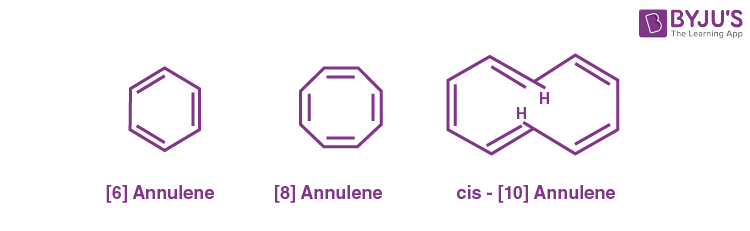Reason R: Planarity is one, of the requirements of aromatic systems.

In the light of the above statements, choose the most appropriate answer from the options given below.

(A) Both A and R are correct and R is the correct explanation of A

(B) Both A and R are correct but R is NOT the correct explanation of A

(C) A is correct but R is not correct

(D) A is not correct but R is correct

Sol.  Annulene is aromatic because it is planar.

 Annulene and  Annulene are both not aromatic because they are not planar. So, Assertion (A) is not correct.

Reason (R) is correct because planarity is one of the requirements of aromatic system.

15.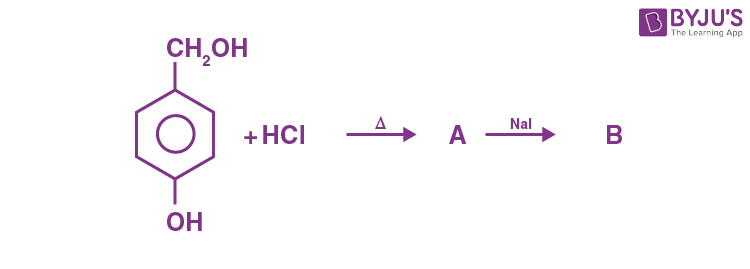In the above reaction product B is:

Product B is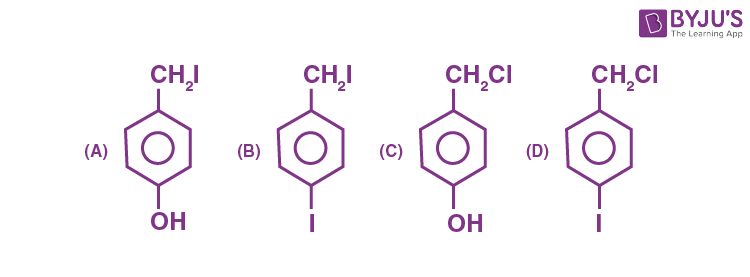Sol.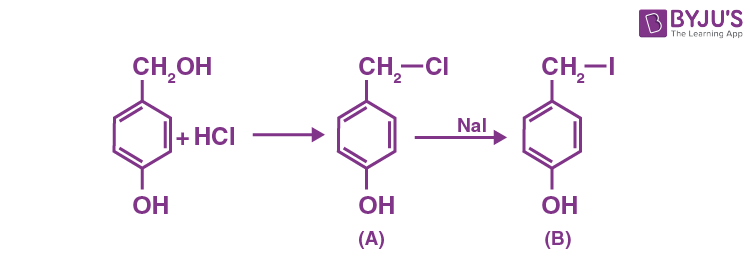Product B is 4-iodomethylphenol.

16. Match List-I with List-II.

List-1

Polymers

List II

Commercial names

A. Phenol-formaldehyde resin I. Glyptal
B. Copolymer of 1,3-butadiene and styrene II. Novolac
C. Polyester of glycol and phthalic acid III. Buna-S
D. Polyester of glycol and terephthalic acid IV. Dacron

Choose the correct answer from the option given below:

(A) A-II, B-III, C-IV, D-I

(B) A-II, B-III, C-I, D-IV

(C) A-II, B-I, C-III, D-IV

(D) A-III, B-II, C-IV, D-I

Sol.

Polymers Commercial names
A. Phenol-formaldehyde resin II. Novolac
B. Copolymer of 1,3-butadiene and styrene III. Buna-S
C. Polyester of glycol and phthalic acid I. Glyptal
D. Polyester of glycol and terephthalic acid IV. Dacron
• The Correct match is

A – II; B – III, C – I ; D – IV

17. A sugar ‘X’ dehydrates very slowly under acidic condition to give furfural which on further reaction with resorcinol gives the coloured product after sometime. Sugar ‘X’ is

(A) Aldopentose

(B) Aldotetrose

(C) Oxalic acid

(D) Ketotetrose

Sol.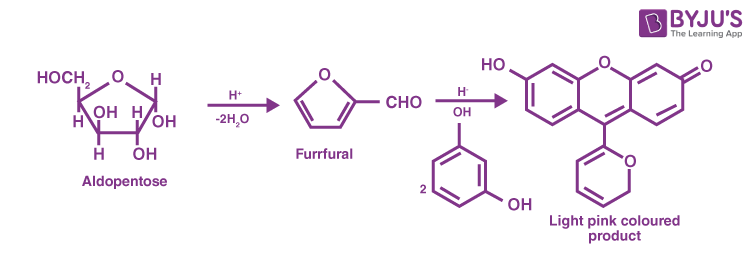This is based on Seliwamoff’s test, which is used to distinguish between aldoses and Kotoses. Ketoses give this test more rapidly than aldoses because they are more rapidly dehydrated than aldoses.

18. Match List I and List II.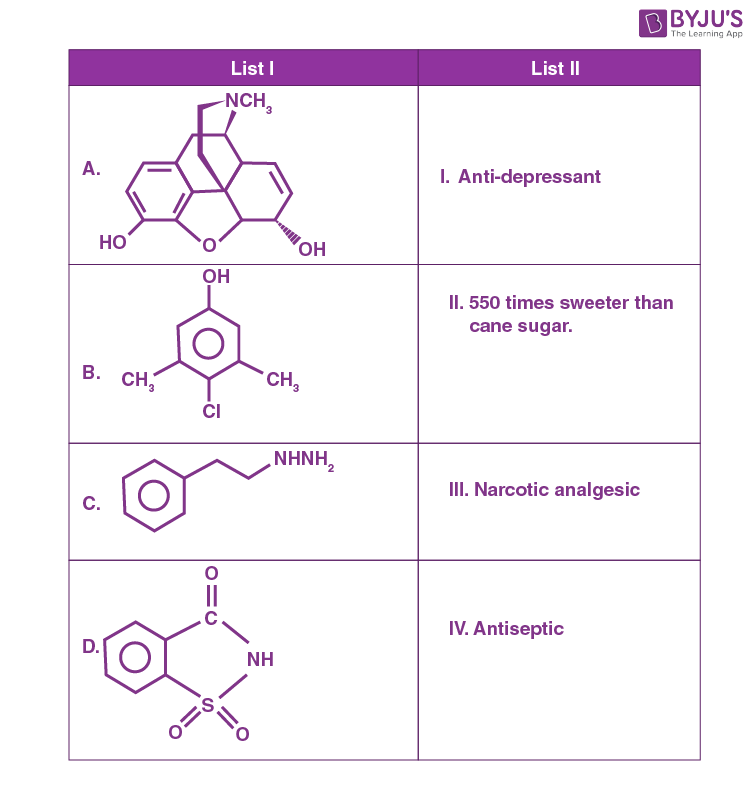Choose the correct answer from the options given below:

(A) A-IV, B-III, C-II, D-I

(B) A-III, B-I, C-II, D-IV

(C) A-III, B-IV, C-I, D-II

(D) A-III, B-I, C-IV, D-II

Sol. • A is morphine which is a narcotic analgesic.

• B is chloroxylenol, an antiseptic.

• C is Nardil, an antidepressant.

• D is saccharin, which is around 550 times sweeter than cane sugar.

19. In Carius method of estimation of halogen, 0.45 g of an organic compound gave 0.36 g of AgBr. Find out the percentage of bromine in the compound.

(Molar masses: AgBr = 188 g mol–1; Br = 80 g mol–1)

(A) 34.04%

(B) 40.04%

(C) 36.03%

(D) 38.04%

Sol.

$$\begin{array}{l}188 ~\text{g of AgBr =} 80 ~\text{g of Br}\\0.36 \text{ g of } AgBr = \frac{80}{188}\times 0.36 \end{array}$$
$$\begin{array}{l}\text{Percentage of Br in given organic compound}\\=\frac{80 \times 0.36}{188 \times 0.45} \times 100= 34.04~\%\end{array}$$

20. Match List I with List II.

List-I List-II
A. Benzene sulphonyl chloride I. Test for primary amines
B. Hoffmann bromamide reaction II. Anti Saytzeff
C. Carbylamine reaction III. Hinsberg reagent
D. Hoffmann orientation IV. Known reaction of Isocyamates

Choose the correct answer from the options given below:

(A) A-IV, B-III, C-II, D-I

(B) A-IV, B-II, C-I, D-II

(C) A-III, B-IV, C-I, D-II

(D) A-IV, B-III, C-I, D-II

Sol. (A) Benzene sulphonyl chloride is also known as Hinsberg reagent.

(B) Hoffmann bromamide reaction involves conversion of amide to amine having one C-atom less. This reaction involves isocyanate as intermediate.

(C) Carbylamine reaction is a test given by all primary amines.

(D) Hoffmann orientation refers to the addition of molecules to unsymmetrical alkenes according to anti Saytzeff’s rule.

Correct match is

A – III; B – IV; C – I; D – II

SECTION – B

Numerical Value Type Questions: This section contains 10 questions. In Section B, attempt any five questions out of 10. The answer to each question is a NUMERICAL VALUE. For each question, enter the correct numerical value (in decimal notation, truncated/rounded-off to the second decimal place; e.g. 06.25, 07.00, –00.33, –00.30, 30.27, –27.30) using the mouse and the on-screen virtual numeric keypad in the place designated to enter the answer.

1. 20 mL of 0.02 M K2Cr2O7 solution is used for the titration of 10 mL of Fe2+ solution in the acidic medium. The molarity of Fe2+ solution is ______×10–2 M. (Nearest integer)

Sol. Applying the law of equivalence,

milliequivalents of Fe2+ = milliequivalents of K2Cr2O7

10 × 1 × M = 20 × 6 × .02

M = 24 × 10–2 M

2. 2NO + 2H2 → N2 + 2H2O

The above reaction has been studied at 800 °C. The related data is given in the table below.

Reaction serial number Initial Pressure of H2/kPa Initial Pressure of NO/kPa Initial rate (-dp/dt_ /(kPa/s)
1 65.6 40.0 0.135
2 65.6 20.1 0.033
3 38.6 65.6 0.214
4 19.2 65.6 0.106

The order of the reaction with respect to NO is ___.

Sol. Let the rate of reaction (r) is as

$$\begin{array}{l} r = K\left[NO\right]^n \left[H_2\right]^m \end{array}$$

From 1st data

0.135 = Kn⋅(65.6)m …(1)

From 2nd data

0.033 = K(20.1)n⋅(65.6)m …(2)

On dividing equation (1) by equation (2)

$$\begin{array}{l}\frac{0.135}{0.033}= \left( \frac{40}{20.1}\right)^n\end{array}$$
$$\begin{array}{l}4 = \left(2\right)^n\\ \therefore n = 2\\ \therefore \text{Order of reaction w.r.t. } NO ~\text{is}~ 2.\end{array}$$

3. Amongst the following, the number of oxide(s) which are paramagnetic in nature is

Na2O, KO2, NO2, N2O, ClO2, NO, SO2, Cl2O

Sol. Paramagnetic species: KO2, NO2, ClO2, NO

Diamagnetic species are : Na2O, N2O, SO2, Cl2O

∴ There are total four paramagnetic molecules.

4. The molar heat capacity for an ideal gas at constant pressure is 20.785 J K–1 mol–1. The change in internal energy is 5000 J upon heating it from 300 K to 500 K. The number of moles of the gas at constant volume is _____. (Nearest integer)

$$\begin{array}{l}\left(Given : R = 8.314~ J K^{–1} mol^{–1}\right)\end{array}$$

Sol.

$$\begin{array}{l} C_p = 20.785 \text{J K}^{-1} \text{mol}^{-1} ~\text{and}~ \triangle U = nC_v \triangle T\end{array}$$
$$\begin{array}{l}\therefore nC_v = \frac{5000}{200}=25\end{array}$$

and we know that

Cp – Cv = R

$$\begin{array}{l}20.785 – \frac{25}{n}=8.314\end{array}$$
$$\begin{array}{l}n = \frac{25}{(20.785 – 8.314)}=2\end{array}$$

5. According to MO theory, number of species/ions from the following having identical bond order is ___.

$$\begin{array}{l}CN^-, NO^+, O_2, O_2^{+}, O_2^{2+}\end{array}$$

Sol.

$$\begin{array}{l}CN^-,\ NO^+\ \text{and}\ O_2^{2+}\ \text{have bond order of}\ ‘3’.\end{array}$$

O2 has bond order of 2,

$$\begin{array}{l}O_2^{+}\ \text{has bond order of 2.5}.\end{array}$$

∴ Three species have similar bond order.

6. At 310 K, the solubility of CaF2 in water is 2.34 ×10–3 g/100 mL. The solubility product of CaF2 is _____ × 10–8 (mol/L)3.

(Given molar mass : CaF2 = 78 g mol–1)

Sol.

$$\begin{array}{l}CaF_2 \overset{s}{\rightleftharpoons } \underset{s}{Ca^{2+}} + \underset{2s}{2F^-}\end{array}$$
$$\begin{array}{l}K_{sp} = s\left(2s\right)^2 \\ = 4s^3\end{array}$$

Solubility(s) = 2⋅34 × 10–3 g/100 mL

$$\begin{array}{l}=\frac{2.34 \times 10^{-3}\times 10}{78} \text{ mole/lit}\\= 3 \times 10^{–4} ~\text{mole/lit}\end{array}$$
$$\begin{array}{l}\therefore K_{sp} = 4 \times \left(3 \times 10^{-4}\right)^3\\ = 108 \times 10^{-12}\\ = 0.0108 \times 10^{-8} (mole/lit)^3\\ \therefore x \approx 0\end{array}$$

7. The conductivity of a solution of complex with formula CoCl3(NH3)4 corresponds to 1 : 1 electrolyte, then the primary valency of central metal ion is ______.

Sol. In 1 : 1 type of electrolyte the ions have +1 and –1 charge on them

∴ Possible compound is → [Co(NH3)4Cl2]+Cl

Oxidation state of central atom represents the total number of primary valency

∴ Primary valency will be 3.

8. In the titration of KMnO4 and oxalic acid in acidic medium, the change in oxidation number of carbon at the end point is _____.

Sol.

$$\begin{array}{l}16H^+ + 2MnO_4^- + 5C_2O_4^{2-}\longrightarrow 10CO_2 + 2Mn^{2+} + 8H_2O\end{array}$$

During titration of oxalic acid by KMnO4, oxalic acid converts into CO2.

∴ Change in oxidation state of carbon = 1

9. Optical activity of an enantiomeric mixture is +12.6° and the specific rotation of (+) isomer is +30°. The optical purity is _______%.

Sol.

$$\begin{array}{l}\text{Optical purity } = \frac{\text{Total rotation}}{\text{specific rotation}}\times 100\end{array}$$
$$\begin{array}{l}=\frac{12.6}{30}\times 100 = 42 \% \end{array}$$

10. In the following reaction,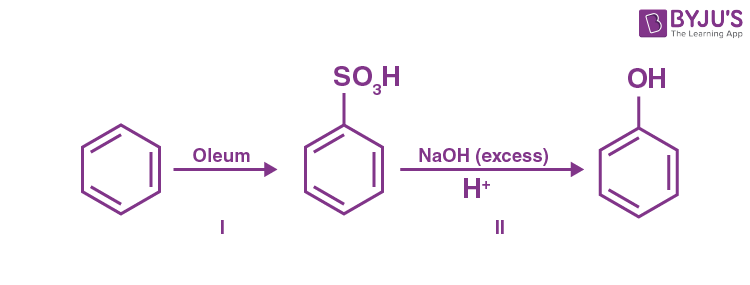the % yield for reaction I is 60% and that of reaction II is 50%. The overall yield of the complete reaction is _______%. [Nearest integer]

Sol.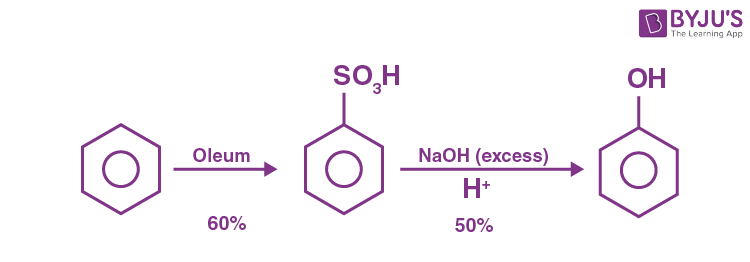The % yield of the complete reaction is

⇒ 0⋅6 × 0⋅5 × 100 = 30%

### Download PDF of JEE Main 2022 July 27 Shift 1 Physics Paper & Solutions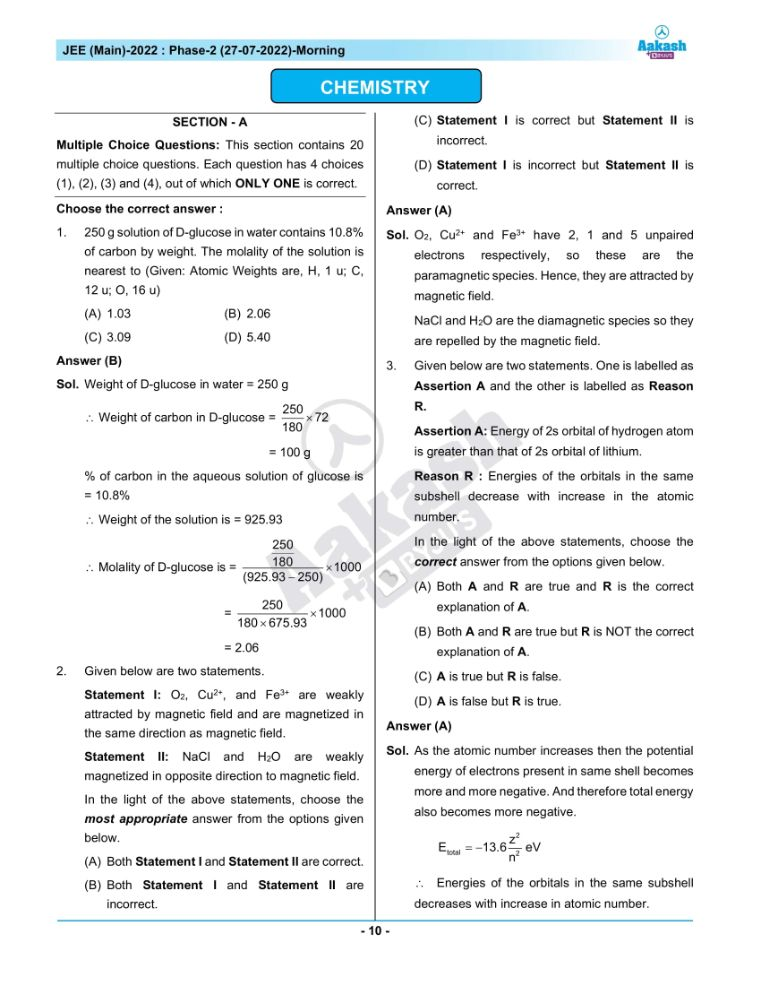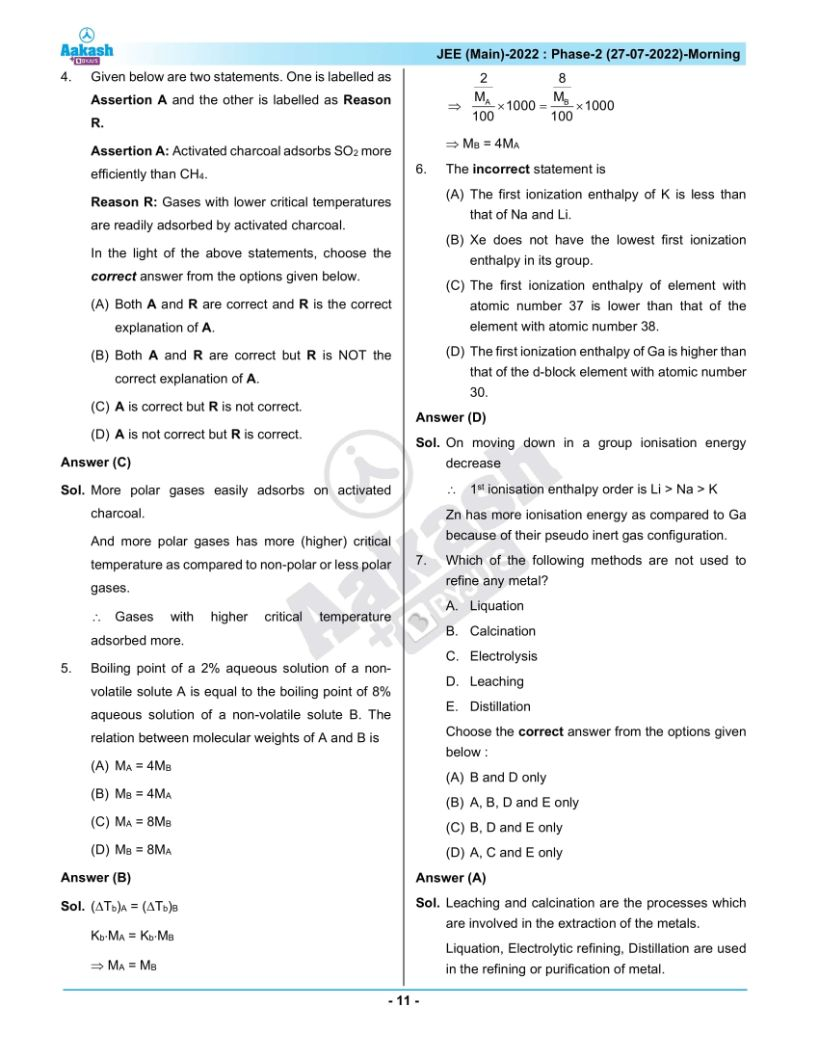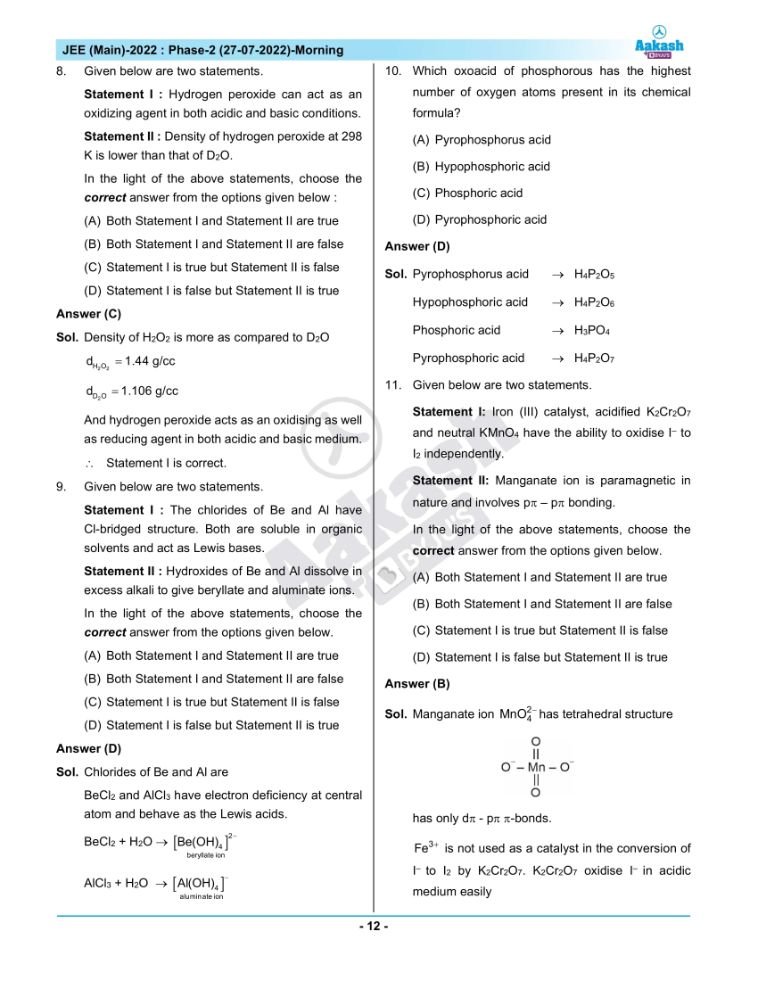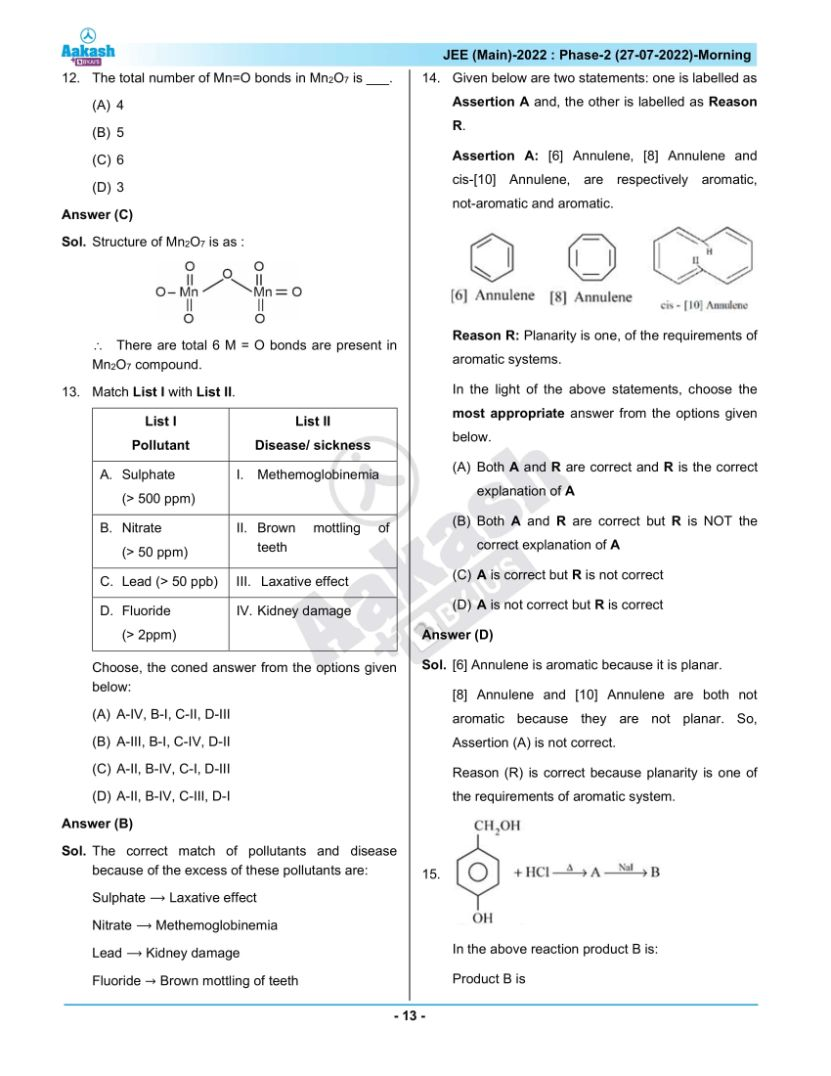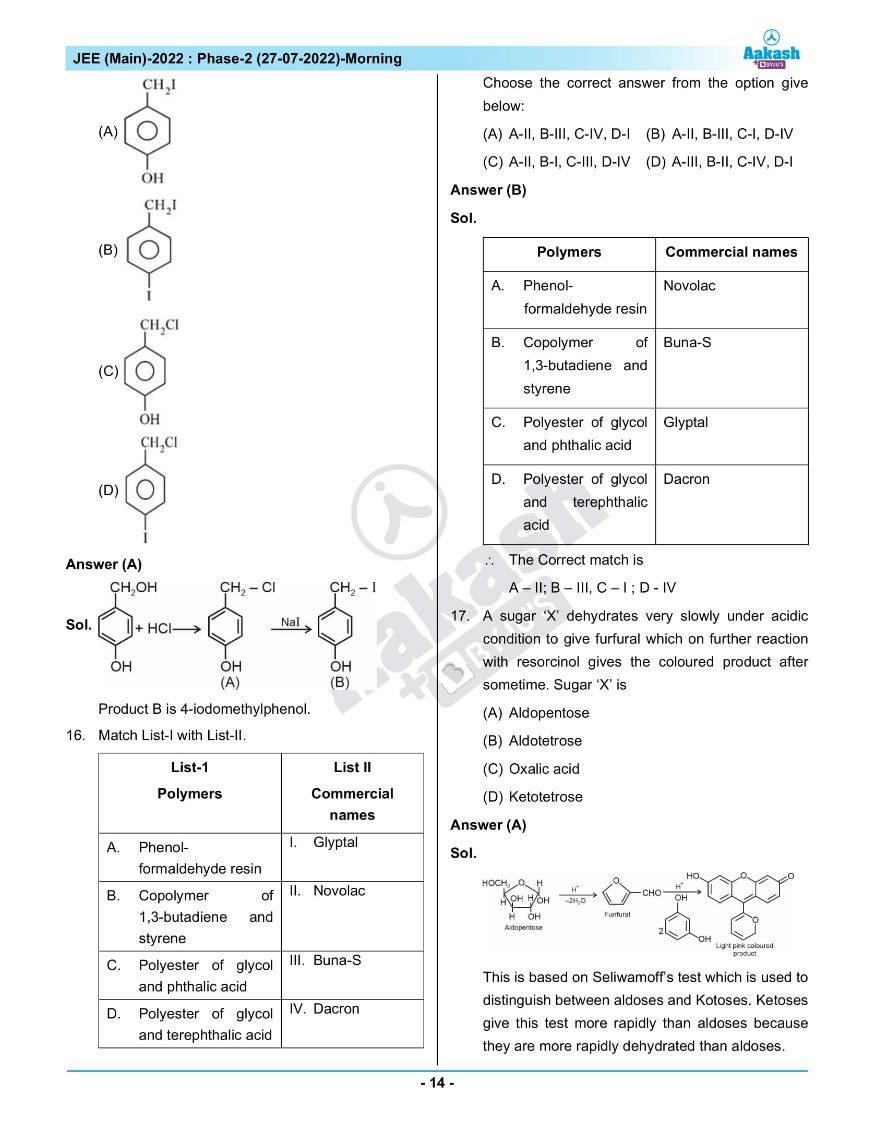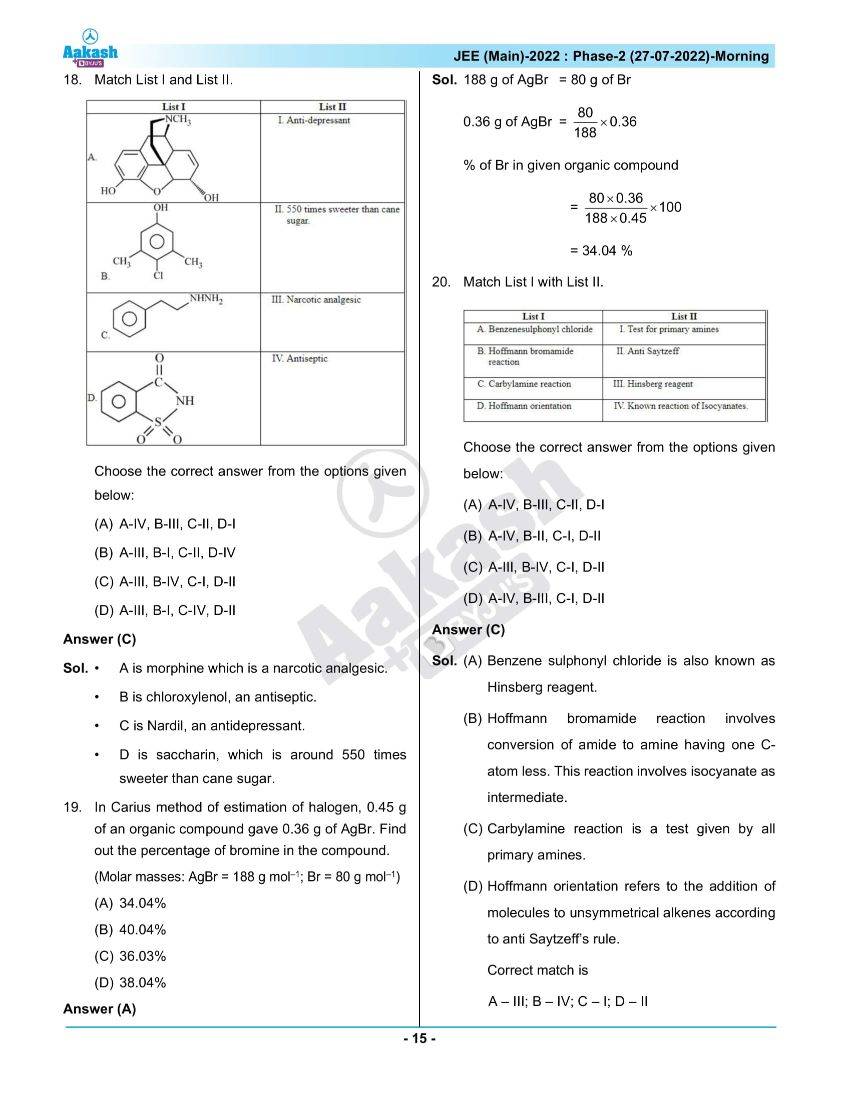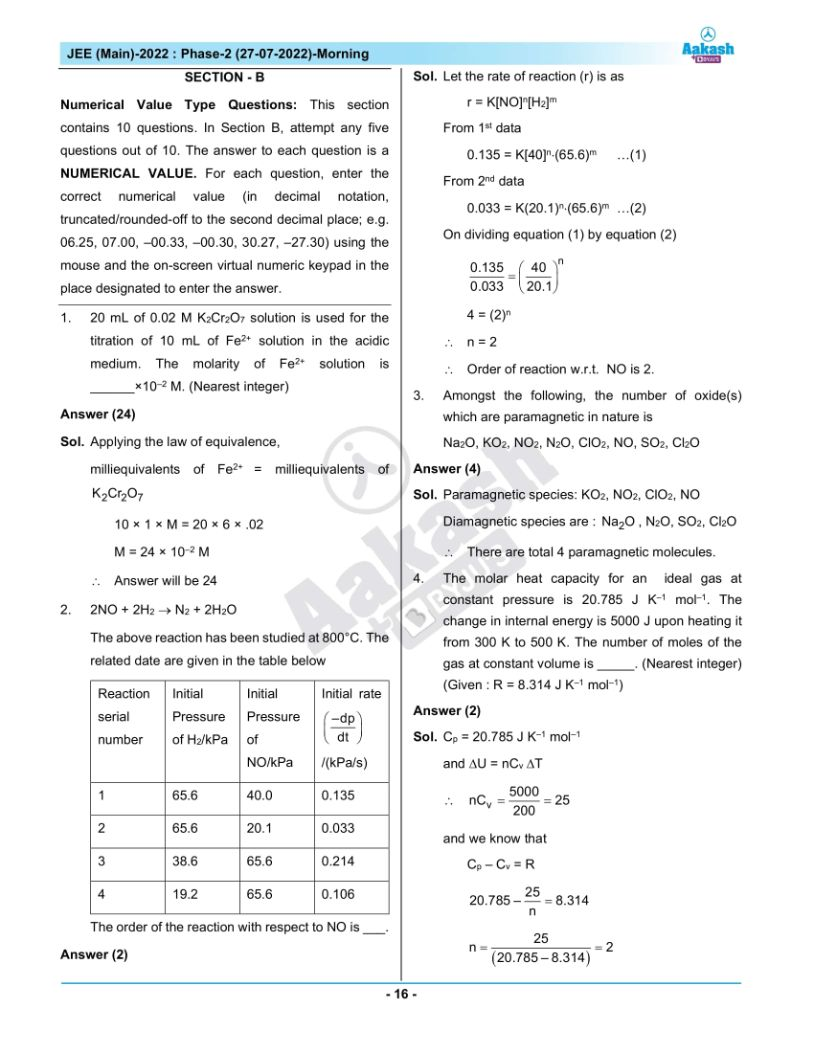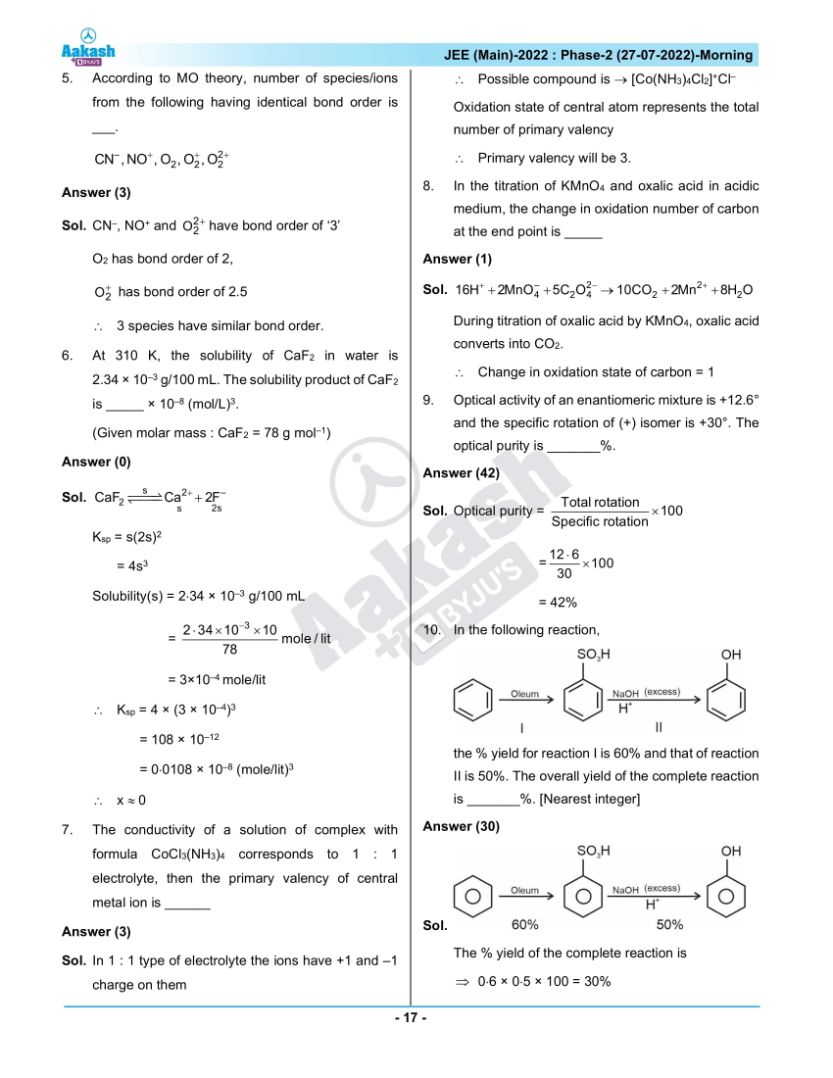## JEE Main 2022 July 27th Shift 1 Paper Analysis